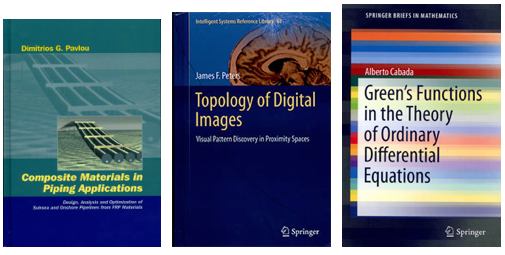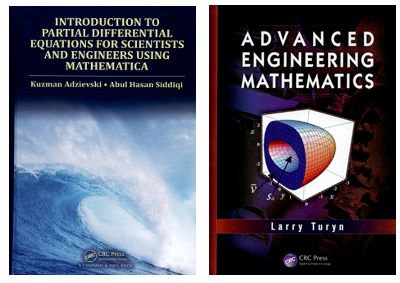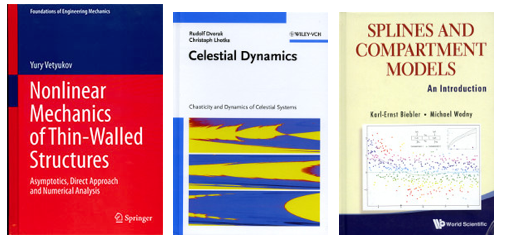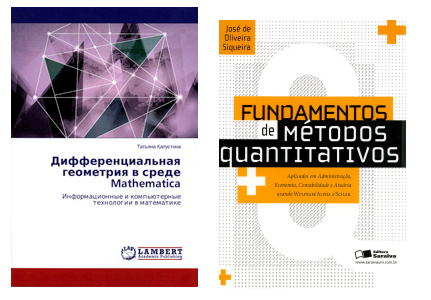Browse by Topic
Related Topics

# New Books Using Wolfram Technologies

Mathematica and Wolfram|Alpha are powerful technologies for deepening students’ and researchers’ understanding of complex topics in math and science. These recently published books incorporate engaging Mathematica and Wolfram|Alpha examples and code into texts written for undergraduates, graduate students, and researchers.Composite Materials in Piping Applications: Design, Analysis and Optimization of Subsea and Onshore Pipelines
Dimitrios G. Pavlou explains the design, analysis, and performance of composite materials in oil, gas, water, and waste-water piping. The text is accompanied by a CD-ROM containing algorithms for pipe design and analysis using Mathematica.

Topology of Digital Images: Visual Pattern Discovery in Proximity Spaces
James F. Peters carries forward recent work on visual patterns and structures in digital images and introduces a near set-based topology in this book. To provide a better understanding of digital images, Mathematica scripts are used to illustrate the fabric and essential features of images.

Green’s Functions in the Theory of Ordinary Differential Equations
In this text written for graduate students and researchers interested in the theoretical underpinnings of boundary value problem solutions, Alberto Cabada provides a complete and exhaustive study of Green’s functions, including two appendices with Mathematica content.Introduction to Partial Differential Equations for Scientists and Engineers Using Mathematica
Written by Kuzman Adzievski and Abul Hasan Siddigi, this textbook gives a mathematical introduction to PDEs at the undergraduate level. The authors use Mathematica along with graphics to improve understanding and interpretation of concepts.

Advanced Engineering Mathematics
This book by Larry Turyn provides accessible and comprehensive mathematical preparation for advanced undergraduate and beginning graduate students taking engineering courses. It includes an online student manual with Mathematica solutions.Nonlinear Mechanics of Thin-Walled Structures: Asymptotics, Direct Approach and Numerical Analysis
Yury Vetyukov presents a hybrid approach to the mechanics of thin bodies. Analytical conclusions and closed-form solutions of particular problems are validated against numerical results. The majority of the book’s simulations were performed in the Wolfram Mathematica environment, and compact source code is included.

Celestial Dynamics: Chaoticity and Dynamics of Celestial Systems
Rudolph Dvorak and Christoph Lhotka convey the sophisticated tools needed to calculate exo-planet motion and interplanetary space flight using Mathematica. The book includes supplements for practical use of formulas.

Splines and Compartment Models: An Introduction
Karl-Ernst Biebler and Michael Wodny present methods of mathematical modeling from two points of view. Splines provide a general approach, while compartment models serve as examples for context related to modeling. The book includes 16 Mathematica programs for solving selected problems.Differentsial’naya geometriya v srede Mathematica: Informatsionnye i komp’yuternye tekhnologii v matematike
(Differential Geometry with Mathematica)
This new Russian-language book by Tat’yana Kapustina discusses key problems in differential geometry and provides Mathematica code for their solutions.

Fundamentos Para Cálculo usando Wolfram|Alpha e Scilab
(Foundations of Calculus—Using Wolfram|Alpha and Scilab)
Written in Portuguese, this text by José de Oliveira Siqueira addresses the transition in calculus between secondary and higher education, using Wolfram|Alpha examples throughout.

Fundamentos de Métodos quantitativos, Aplicados em Administracao, Economia, Contabilidade e Atuaria usando Wolfram|Alpha e Scilab
(Foundations of Quantitative Methods in Business Administration, Economics, Accounting and Actuarial Science—Using Wolfram|Alpha and Scilab)
Also by José de Oliveira Siqueira, Foundations of Quantitative Methods presents major mathematical methods applied to various social sciences with Wolfram|Alpha examples.